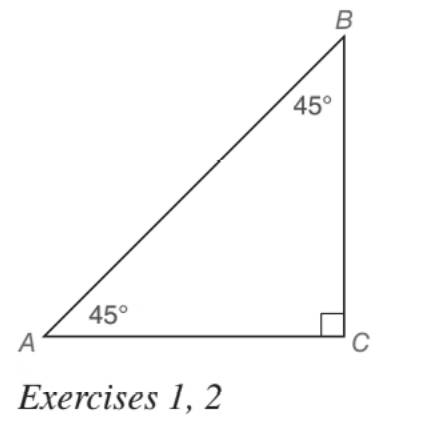Chapter 5.5, Problem 2EElementary Geometry For College St...

7th Edition
Alexander + 2 others
ISBN: 9781337614085

Solutions

Chapter
SectionElementary Geometry For College St...

7th Edition
Alexander + 2 others
ISBN: 9781337614085
Textbook Problem

For the 45 ° - 45 ° - 90 ° triangle shown, suppose that A B = a 2 . Find: a) A C b) B CTo determine

a) To find:

AC, for the 45°-45°-90° triangle shown, suppose that AB=a2.

Explanation

Approach:

For a right triangle for which the measure of the interior angles 45°, 45°, and 90°, if ‘a’ is the length of measure of one of the leg; opposite to the angle 45°, then the length of the other two sides is given by

Length of the other leg =a

Length of the hypotenuse =a2

In general

Length of the legs are equal.

Length of the hypotenuse =2× (Length of one of the legs)

Calculation:

Given,

A 45°-45°-90° triangle ABC with AB=a2.

45°-45°-90° theorem.

In a right triangle whose angle measures 45°, 45°, and 90°, the legs are congruent and the hypotenuse has a length equal to the product of 2 and the length of either leg

To determine

b) To find:

BC, for the 45°-45°-90° triangle shown, suppose that AB=a2.

Still sussing out bartleby?

Check out a sample textbook solution.

See a sample solution

The Solution to Your Study Problems

Bartleby provides explanations to thousands of textbook problems written by our experts, many with advanced degrees!

Get Started

Solve each inequality for x. 55. (a) ln x 0 (b) ex 5

Single Variable Calculus: Early Transcendentals, Volume I

26. Write the first seven terms of the sequence defined by .

Mathematical Applications for the Management, Life, and Social Sciences

In Exercises 49-62, find the indicated limit, if it exists. 61. limx1x1x3+x22x

Applied Calculus for the Managerial, Life, and Social Sciences: A Brief Approach

Expand each expression in Exercises 122. (x32x2+4)(3x2x+2)

Finite Mathematics and Applied Calculus (MindTap Course List)

Sometime, Always, or Never:

Study Guide for Stewart's Single Variable Calculus: Early Transcendentals, 8th